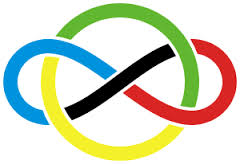USAMO is filled with Vieta

Algebra Level 4The product of two of the four zeros of the quartic equation $x^4 - 18x^3 + kx^2 + 200x - 1984 = 0$ is -32. Find $k$.

×